# Sapphire: Efficient prototyping of recurrences

## Magne Haveraaen

### Abstract

Recurrence relations is the mathematical form used to express many important problems. Being able to rapidly and efficiently prototype a recurrence will aid research and engineering in diverse areas as heat flow, seismic processing, dynamic programming, etc. Unfortunately, the conventional techniques available is either to program the recurrence as a recursive program with exponential growth in time complexity, or unfold the recursion and hand code an efficient solution, with the associated high development costs. Here we present an approach that combines the best of these two methods.

### Background

Computational modelling using partial differential equations (PDEs) is becoming an important tool for industry. Currently this is hampered by the time and effort needed to develop good computational models, and the time and cost needed to port such a model onto a high performance computer (HPC).

Recurrence relations form the core of a computational model of a PDE. For many problems, such as those in computational fluid dynamics, formulating an appropriate recurrence relation is a non-trivial task. Often it will require several iterations of formulating a recurrence, creating a prototype implementation and trying it on selected data sets, before a usable strategy is found. The test problems may require the use of an HPC to increase the turn-around time during the computational model development cycle. Being able to rapidly prototype reasonably efficient implementation of recurrence relations will significantly reduce the time and effort needed to find the appropriate recurrence.

### Recurrence relations

An order k linearly dependent recurrence r with natural numbers as index domain is given by the form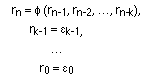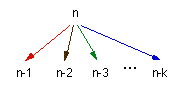The picture illustrates the dependency of step n of the recurrence on steps n, n-1, .., n-k. For n<k base values are given as constants. A standard example of an order 2, linearly dependent recurrence is the Fibonacci function, given by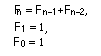It is obvious that this can be expressed as a recursive program
`F(n) = if n > 1 then F(n-1) + F(n-2) else 1`

which, when compiled in a conventional language will give the following execution tree for F(5).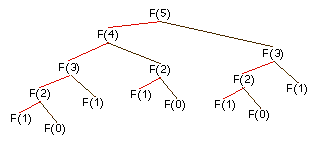Conventional recursive execution

Note how the subtrees for F(3) are duplicated and F(2) are triplicated. This multiplicity of identical subtrees gives an exponential execution time, which is prohibitively expensive even for moderately sized arguments to this simple function. By merging subtrees, we get the much more compact execution graph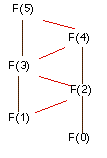Optimal execution strategy

which only grows linearly in size with the argument to the function. This graph is the one we achieve by the normal hand coded version of the Fibonacci function.

### Generalised recurrence relations

The concept of a recurrence relation can be generalised to arbitrary discrete index domains. Given a collection of index variables n, m, ..., j, each ranging over the natural numbers, the generalised recurrence has the form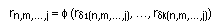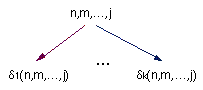where the dependency pattern, often called a stencil, is given by the k expressions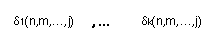each returning a full index set n,m,...,j, and with a suitable collection of initial values. Note that we have to put certain conditions of wellfoundedness on the dependency pattern and choice of initial values in order to make r well defined. Thus one might view the recurrence as composed from three parts: a structural part (the stencil), an expression part (the recursive part of the recurrence definition), and an initialisation part.

The general form of the recurrence relation, encompasses a large class of recursive equations. Some problems include
There is also a theory of executing these programs on parallel machines.

### Prototyping recurrences

The natural way of expressing a recurrence relation in a programming language such as C++ would be as recursive function calls mimicing the recursive equations from the mathematical formulation. Unfortunately, this results in a program growing exponentially both in storage and time usage, while a non-recursive implementation may need only a fixed amount of storage and linear time (as in the case of the Fibonacci relation), or linear growth of storage and `n log n` or ```n (log n)^2``` growth of time as in the FFT and bitonic sorting cases, respectively. Thus naively writing a recurrence as a recursive function is not a suitable path for prototyping recurrences.

The other alternative, hand coding a non-recursive version, gives efficient code, but is a difficult, hence costly, task if the stencil is complex. Thus this is not a viable approach for prototyping recurrences.

Our approach is to use the recursive formulation of the recurrence, but augment the definition of the stencil D, with the definition of the opposite of the stencil, Ð. This allows us to compile the recursively structured recurrence function into non-recursive code, giving the same time and space complexity as one would expect from a reasonably efficient implementation. One can also augment D and Ð with information on the distribution of the computation on the processors of a parallel computer. This information will also define the communication paths, thus yielding full control over the parallel execution of the code.

To improve the usability of this approach to prototyping recurrences, we will also develop a library of DDA's representing different standard stencils. For a given stencil, the library will also include instructions for the parallelisation of the recurrences using it, thus allowing a prototype implementation of a recurrence to be run efficiently on several HPC architectures without requiring the user to rewrite the recurrence program.

### Summary

• Recurrence relations occur in many formulations of common problems
• Partial differential equations
• Dynamic programming
• Fast Fourier transform
• and others
A prototyping tool yielding fairly efficient code would potentially be very useful
• Conventional approaches to programming recurrences include
• Implement a recurrence as a recursive procedure in a conventional language
• straight forward to implement
• exponentially growing execution times unless the recurrence is trivial
Not suited for prototyping: not practical for interesting problems.
• Hand-code a non-recursive solution
• often quite complicated unless the recurrence is trivial
• good execution efficiency
Not suited for prototyping: implementation costs are high
• Efficient prototyping achievable by
• allowing the user to write the recurrence recursively
• explicit definitions of the dependency D and opposite communication Ð
• embedding on parallel machines are definable as program code

### Acknowledgements

Steinar Søreide has produced the HTML-code and moving images for this WWW presentation and prototyped the translation tool that compiles recurrences to efficient programs.

These pages last updated January 1999 ©1997 Magne Haveraaen, University of Bergen, Norway.